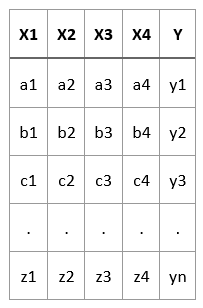Prerequisites to study: Introduction to Machine Learning

In Supervised Learning, the data comprises of inputs and the corresponding output. Using this the machine learning system will build the model so that given a new observation X, it will try to find out what is the corresponding y (output). So in Supervised Learning for every instance, we tell what is the output. Such type of instances or data is also known as labeled data.

In Supervised Learning, we have a set of input features, X1, X2, X3, …. Xn for which we describe the instances and we have a target feature Y.

There are several instances and each instance comprises the values of the features.

In the following data, we have a number of instances X1<a1,a2,a3,a4>, X2<b1,b2,b3,b4> and so on.

The target feature in the dataset is given as Y<y1,y2,y3,….,yz>

Therefore, the instances are described in terms of features. There are input features and there is a corresponding output attribute.In Supervised Learning, we are already provided with this data for training the model. But during the testing time, we are only provided with the input features. The output feature during the testing time is something we need to calculate/predict/output.

The output feature (Y) can be a discrete-valued feature or a continuous-valued feature.

If Y is a discrete-valued feature, we call such a problem as a Classification Problem. For example, Y can signify whether or not it will rain tomorrow. Or given a set of symptoms, predict whether or not the patient has a particular disease. Therefore, Y is a Boolean target vector.

If Y is a continuous-valued feature, we call such a problem as a Regression Problem. For example: Given the values of certain features we want to predict the price of a house. We may be given the location of the house, the floor area of the house, the number of bedrooms in the house, and using these features we want to predict the price of the house which is a continuous-valued or a real value number.

Example of classification problems –

• Credit Scoring: Given the income and the savings of a person predict if it a high-risk person or a low-risk person.

Example of Regression Problem –

• Price of a used car: Given the mileage of a car predict the price of the car.